Math Courses / Course / Chapter

# Convergence vs. Divergence of a Series

Cynthia Helzner, Christopher Haines, Kathryn Boddie
• Author
Cynthia Helzner

Cynthia Helzner has tutored middle school through college-level math and science for over 20 years. She has a B.S. in microbiology from The Schreyer Honors College at Penn State and a J.D. from the Dickinson School of Law. She also taught math and test prep classes and volunteered as a MathCounts assistant coach.

• Instructor
Christopher Haines
• Expert Contributor
Kathryn Boddie

Kathryn has taught high school or university mathematics for over 10 years. She has a Ph.D. in Applied Mathematics from the University of Wisconsin-Milwaukee, an M.S. in Mathematics from Florida State University, and a B.S. in Mathematics from the University of Wisconsin-Madison.

Convergence and divergence of a series in math follows some specific rules. Learn the rules as well as the geometric series convergence test. Also see examples. Updated: 03/15/2022

Show
• FAQs
• Activities

In the following examples, students will practice determining whether a Geometric Series converges or diverges and find what the Geometric Series converges to; if it does converge. The examples start as very basic and develop to involving more algebraic manipulation as the student progresses. After completing the examples, students will be better able to recognize a geometric series and how to determine its convergence.

## Examples

Recall that a geometric series of the form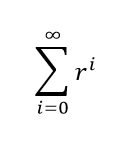converges to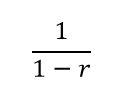if |r|<1. In general, if "a" is a constant, then a geometric series of the form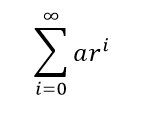converges to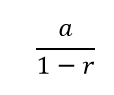if |r|<1. Determine if the following series converge or diverge - and if they converge, find the value the series converges to.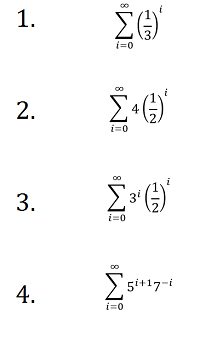## Solutions

1. Since we have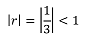the geometric series converges. It converges to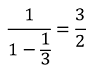2. Since we have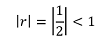the geometric series converges. It converges to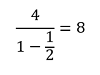3. We need to rewrite this series so that it is in the proper form. We have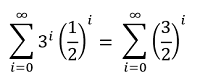Then, since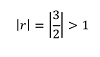the geometric series diverges.

4. We need to rewrite this series so that it is in the proper form. We have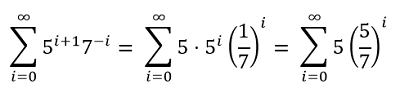Then, since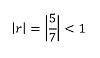the geometric series converges. It converges to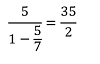#### What is convergence and divergence series?

A series is the sum of a sequence of numbers. A convergent series is a series that approaches a set limit, while a divergent series is a series that does not approach a set limit.

#### How do you know if its convergence or divergence?

Pick a convergence or divergence test based on the type of series. For example, to test a geometric series, see if |r| < 1. If so, the geometric series converges; if not, it diverges. In contrast, an arithmetic series always diverges.

#### What does a convergent geometric series mean?

A geometric series is the sum of a sequence of numbers in which each term is multiplied by the same number, r, to produce the next term. A convergent geometric series is a geometric series that approaches a set limit.

#### How do you know if a geometric series converges?

If |r| < 1, the absolute values of the terms get smaller, so the geometric series converges. The quantity r is the common ratio, i.e., what each term is multiplied by to produce the next term.

A series is the sum of a sequence, which is a list of numbers that follows a pattern. An infinite series is the sum of an infinite number of terms in a sequence, such as 2 + 4 + 6 + 8 + ... Infinite series are subject to convergence and divergence.

In Zeno's Paradox of the Dichotomy, a runner must always reach the halfway point between her current position and the finish line. Those distances will become half as large each time. Thus, there will always be some distance left to go, and so she will never reach her destination. This is similar to a car that reduces its speed by half each second so that it will travel half of the prior second's distance. Like the runner, the car will never reach its destination. In both of these cases, the distances traveled become smaller by half each time, and so the series of distances traveled converges to a set limit (the total distance of the trip).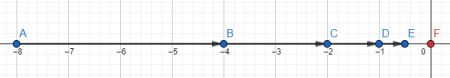To unlock this lesson you must be a Study.com Member.

## Convergence/Divergence Series

Let's say a traveler is driving a car down a road that is one mile long. At the start, the car is moving 60 miles per hour. After one second, the car immediately reduces its speed to 30 miles per hour. After two seconds, the car instantaneously reduces its speed from 30 miles per hour to 15 miles per hour. More generally if v(t) is the speed of the car at time t, then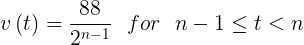This is where n = 1, 2, 3, 4, 5,......

In the formula, the time t is in seconds, and the speed v(t) is in feet per second, and vn is the distance traveled after n seconds.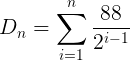The distance traveled after n seconds takes the form of a geometric sum. This sum simplifies to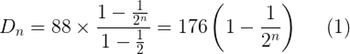By passing this equation, which we've called (1), to the limit as n tends to infinity, we can then see that the car's distance will approach 176 feet as the time t tends to infinity. However since the expression given in (1) is strictly less than 176, the car will never reach the end of the road. Instead, the car slows down so drastically that it gravitates towards the 176 foot marker. The marker is seen as a point of attraction along the one mile road. It appears counter-intuitive that even though the car never reduces its speed to zero, it never comes anywhere close to reaching the end of the road - it's not even one-thirtieth of the distance!

Figure 1 illustrates the car's traveling progression. The first car represents the position at zero seconds, the second car at one second, the third at two seconds, and so on (from left to right). As is apparent, the one second increments decrease with time. We can also see this from the identity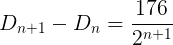The moral of this story is that a sum having an infinite number of terms can possibly end up being finite. We've given an example of a convergent geometric series, making the concept of a convergent series more precise.An error occurred trying to load this video.

Try refreshing the page, or contact customer support.

Coming up next: Repeating Decimals as Series

### You're on a roll. Keep up the good work!

Replay
Your next lesson will play in 10 seconds
• 0:04 Convergence/Divergence Series
• 2:38 The Theorem
• 6:20 Lesson Summary
Save Timeline
Autoplay
Autoplay
Speed Speed

There are a variety of convergence theorems and tests for a variety of series types. In essence, any convergence theorem states that if a series approaches a high or low limit, it converges.

To unlock this lesson you must be a Study.com Member.

Convergence tests are used to determine whether a series will converge. The particular test to use varies based on the characteristics of the series being analyzed, but, in general, the tests determine whether the sum of the terms approaches a finite limit. Some tests do this by determining whether the absolute values of the terms get smaller, while others use limits and integrals from calculus.

### Geometric Series Convergence Test

The geometric series convergence formula is {eq}\frac{a}{1-r} {/eq} if |r| < 1, where a is the first term and r is the common ratio, i.e., the number that each term is multiplied by in order to produce the next term.

To unlock this lesson you must be a Study.com Member.

Before looking at some convergent series examples, consider what makes a series convergent:

• The sum of the terms approaches (but does not reach) a set limit.
• The absolute values of the terms get smaller.
• The terms get closer to zero.
• For a geometric series, |r| < 1.

### Geometric Series Convergence

A geometric series written in standard form is {eq}\displaystyle\sum_{n=1}^{\infty}ar^{n-1} {/eq} or {eq}\displaystyle\sum_{n=1}^{\infty}ar^{n} {/eq}. For a geometric series written in standard form, the base of the exponent is r so, if the base of the exponent is less than 1, then |r| < 1 and the series will converge. If the geometric series is not written in standard form, plug-in two consecutive values of n and divide the second term by the first term to get r.

To unlock this lesson you must be a Study.com Member.

All that is required for a series to be divergent is for it to not approach a set limit. Thus, any of the following would make a series divergent:

• The sum of the terms does not approach a set limit.
• The sum of the terms alternates between two set values.
• The sum of the terms approaches infinity or negative infinity.
• The absolute values of the terms get larger.
• The terms get further from zero.
• For a geometric series, {eq}|r| \geq 1 {/eq}.

To unlock this lesson you must be a Study.com Member.

A series is the sum of a sequence, and an infinite series is the sum of an infinite sequence. Some infinite series converge to a finite limit. Those that do not are said to diverge. The Geometric Series Theorem gives the values of the common ratio, r, for which the series converges and diverges: a geometric series will converge if r is between -1 and 1; otherwise, it will diverge. If the series converges, then the infinite sum is {eq}\frac{a}{1-r} {/eq}, where a is the first term and r is the common ratio. To find a, plug in the first value of the variable into the term formula. In sigma notation, the term formula is what is written after the sigma, the variable is the letter before the = sign under the sigma, and the first value of the variable is what is written after the = sign under the sigma.

Just as series can converge or diverge, functions can converge or diverge as well. A convergent function is a function that approaches a set limit (i.e., it has a horizontal asymptote). A divergent function is a function that does not approach a set limit (i.e., no horizontal asymptote). Divergent series and functions often approach positive or negative infinity, but they need not do so; any series or function that fails to converge to a finite limit is divergent.

To unlock this lesson you must be a Study.com Member.

## The Theorem

To begin, we define the theorem, which we'll call equation 2: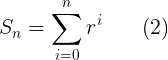where r does not equal 0 if the limit of a partial sum exists as a real number. We write the definition of an infinite series, like this one, and say the series, like the one here in equation 3, converges.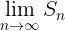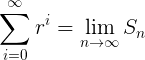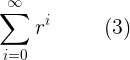If the aforementioned limit fails to exist, the very same series diverges. It's denoted as an infinite sum whether convergent or divergent.

The partial sums in equation 2 are geometric sums, and this is because the underlying terms in the sums form a geometric sequence. The respective infinite series is then called a geometric series.

Which values of r does the series given in (3) converge? It turns out that there is a convenient, shortcut formula for the partial sums defined in (2). The formula can be proven directly by induction, and for that reason, we omit the proof here.

Here's the Lemma: Geometric Sum Formula in equation 4:

For n = 0, 1, 2, 3, 4, ....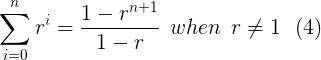As an immediate consequence of equation 4, we arrive at the geometric series theorem. This theorem gives the value of r for which the series converges and diverges.

We first let the partial sums be defined as in (2) and the respective infinite series defined as in (3). Then, we have the following results: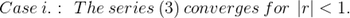In this case, we get our geometric series formula and case ii: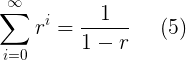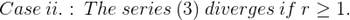In this second case, we get the following resulting sum value in case ii and the divergent oscillation in case iii.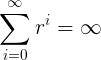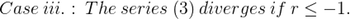In this third and final case, the series cannot be assigned any value, since the limit of the partial sums does not exist even in the extended real number sense. The monotone convergence theorem ensures the existence of the limits in cases i and ii (as extended real numbers).

Video Transcript

## Convergence/Divergence Series

Let's say a traveler is driving a car down a road that is one mile long. At the start, the car is moving 60 miles per hour. After one second, the car immediately reduces its speed to 30 miles per hour. After two seconds, the car instantaneously reduces its speed from 30 miles per hour to 15 miles per hour. More generally if v(t) is the speed of the car at time t, thenThis is where n = 1, 2, 3, 4, 5,......

In the formula, the time t is in seconds, and the speed v(t) is in feet per second, and vn is the distance traveled after n seconds.The distance traveled after n seconds takes the form of a geometric sum. This sum simplifies toBy passing this equation, which we've called (1), to the limit as n tends to infinity, we can then see that the car's distance will approach 176 feet as the time t tends to infinity. However since the expression given in (1) is strictly less than 176, the car will never reach the end of the road. Instead, the car slows down so drastically that it gravitates towards the 176 foot marker. The marker is seen as a point of attraction along the one mile road. It appears counter-intuitive that even though the car never reduces its speed to zero, it never comes anywhere close to reaching the end of the road - it's not even one-thirtieth of the distance!

Figure 1 illustrates the car's traveling progression. The first car represents the position at zero seconds, the second car at one second, the third at two seconds, and so on (from left to right). As is apparent, the one second increments decrease with time. We can also see this from the identityThe moral of this story is that a sum having an infinite number of terms can possibly end up being finite. We've given an example of a convergent geometric series, making the concept of a convergent series more precise.

## The Theorem

To begin, we define the theorem, which we'll call equation 2:where r does not equal 0 if the limit of a partial sum exists as a real number. We write the definition of an infinite series, like this one, and say the series, like the one here in equation 3, converges.If the aforementioned limit fails to exist, the very same series diverges. It's denoted as an infinite sum whether convergent or divergent.

The partial sums in equation 2 are geometric sums, and this is because the underlying terms in the sums form a geometric sequence. The respective infinite series is then called a geometric series.

Which values of r does the series given in (3) converge? It turns out that there is a convenient, shortcut formula for the partial sums defined in (2). The formula can be proven directly by induction, and for that reason, we omit the proof here.

Here's the Lemma: Geometric Sum Formula in equation 4:

For n = 0, 1, 2, 3, 4, ....As an immediate consequence of equation 4, we arrive at the geometric series theorem. This theorem gives the value of r for which the series converges and diverges.

We first let the partial sums be defined as in (2) and the respective infinite series defined as in (3). Then, we have the following results:In this case, we get our geometric series formula and case ii:In this second case, we get the following resulting sum value in case ii and the divergent oscillation in case iii.In this third and final case, the series cannot be assigned any value, since the limit of the partial sums does not exist even in the extended real number sense. The monotone convergence theorem ensures the existence of the limits in cases i and ii (as extended real numbers).

To unlock this lesson you must be a Study.com Member.

### Register to view this lesson

Are you a student or a teacher?

Back

### Resources created by teachers for teachers

Over 30,000 video lessons & teaching resources‐all in one place.Video lessonsQuizzes & WorksheetsClassroom IntegrationLesson Plans

I would definitely recommend Study.com to my colleagues. It’s like a teacher waved a magic wand and did the work for me. I feel like it’s a lifeline.

Jennifer B.
TeacherCreate an account to start this course today
Used by over 30 million students worldwide